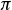It is given that sin A = K , where A is an angle measured in radians and (pi)< A < 3(pi)/2 . If sin B = K which of the following could be the value of B ?
A) A – (pi)
B) (pi) + A
C) 2(pi) – A
D) 3(pi) – A

If A is betweenand, then it’s in the 3rd quadrant, which means its sine is negative. So you know is that K is negative.

Knowing that sines are negative in the 3rd and 4th quadrants, which of the answer choices will land you in one of those quadrants, knowing that A is in the 3rd quadrant?

A – π will put you in the 1st quadrant.

π + A will also put you in the 1st quadrant.

2π – A will put you in the 2nd quadrant.

3π – A will put you in the 4th quadrant. That works—the sine is negative there!

If you’re having trouble with these calculations, you might find it useful to plug in a value that works for A, like A = 1.25π. then take the sine of each choice with your calculator to see which one ends up negative.